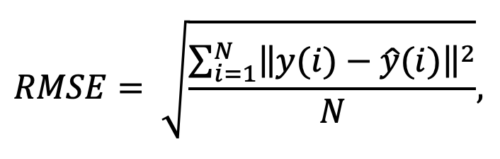Akhil Chaudhary
NIT Warangal graduate, Software Engineer at Wipro.

# Root Mean Squared ErrorThe Root Mean Squared Error (RMSE) is one of the most commonly used measures for evaluating the quality of a predictive model. It shows how far the predicted values are from actual values with respect to the Euclidean distance between them.

RMSE is expressed as:where N is the number of data points, y(i) is the ith measurement, and y ̂(i) is its corresponding prediction.

RMSE is not scale-invariant, and therefore the comparison of models is affected by the scale of the data. Thus, it is advisable to apply RMSE over standardized data.

# CODE

import numpy as np

print(np.sqrt(metrics.mean_squared_error(y_test, y_pred)))

# USES

It is extremely useful to have a single number as an evaluation metric for any model. RMSE is the most widely-used and intuitive scoring rule that is easy to understand. It is also compatible with the majority of statistical techniques.

Absolute RMSE error is of no value on its own for training models. During training, we need a heuristic that helps us decrease the error during each iteration, an indication that the model is improving over iterations.

In evaluating trained models, however, absolute values are important. Now, it is not about whether the model performs better than last time, but whether the model is capable of solving a practical problem. This value can be however small and only depends on how accurate we want the model to be.

# LIMITATIONS & ALTERNATIVES

By squaring errors and calculating mean, RMSE is vulnerable to predictions that are much worse than others, i.e. bad predictions affect the RMSE score heavily. To tackle this, the absolute value of errors can be used (Mean Absolute Error, or MAE). Another approach is to use median values to get a better idea of how the model performs on most predictions without much influence from poor predictions.